# (Solved) : Use Octave Matlab Programs Code Use Disp Num2str Also Use Break Continue Statements Q42705206 . . .

use octave or MATLAB as programs to code. do not usedisp or num2str. also, do not use break or continuestatements.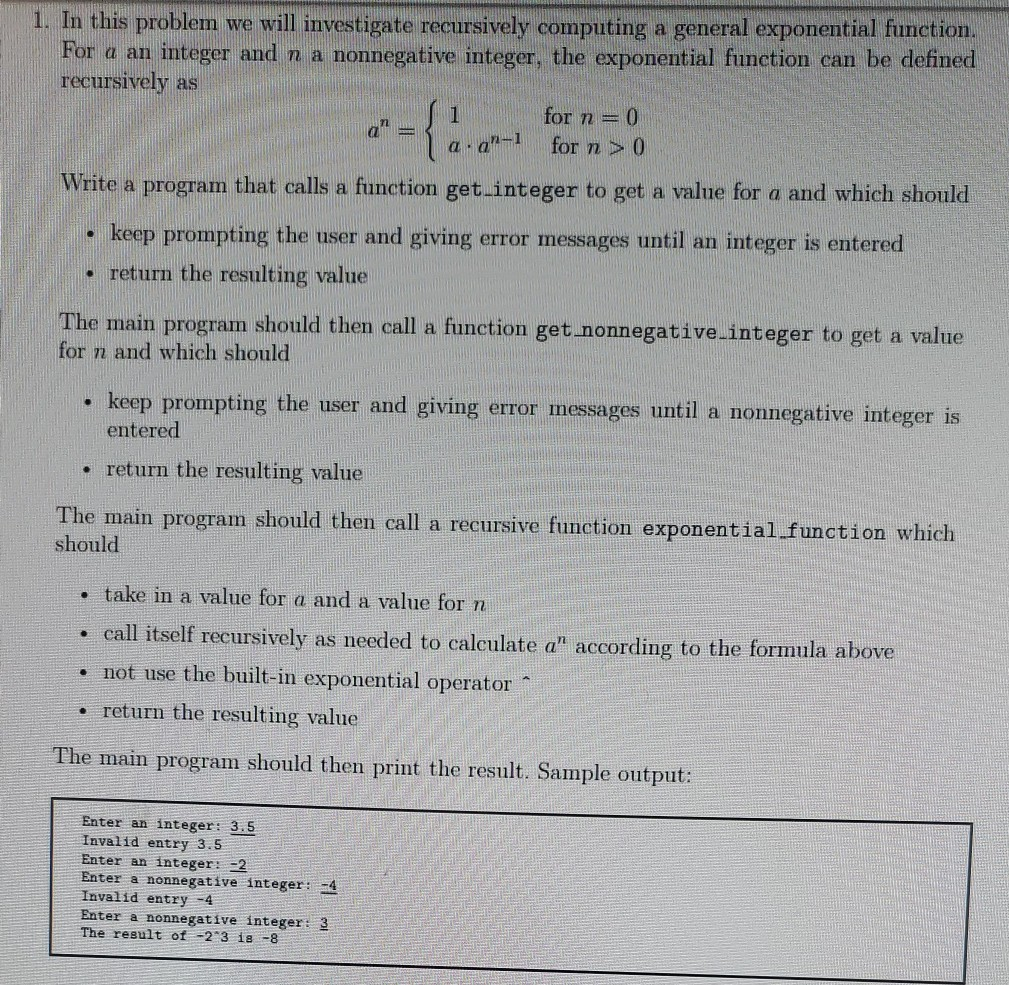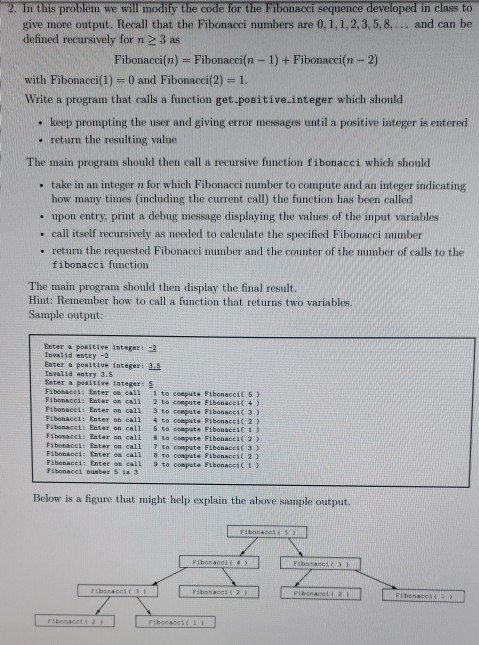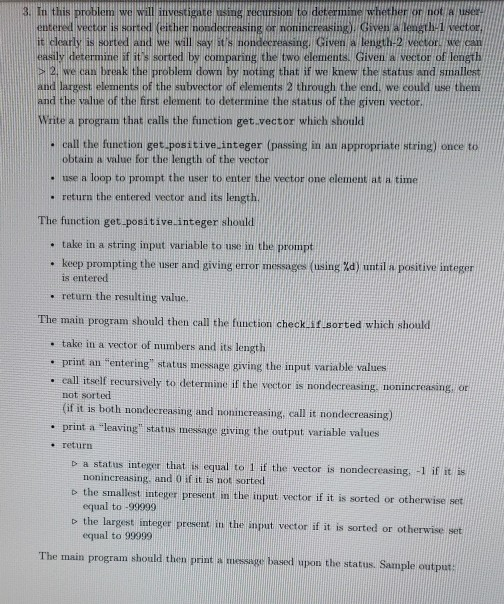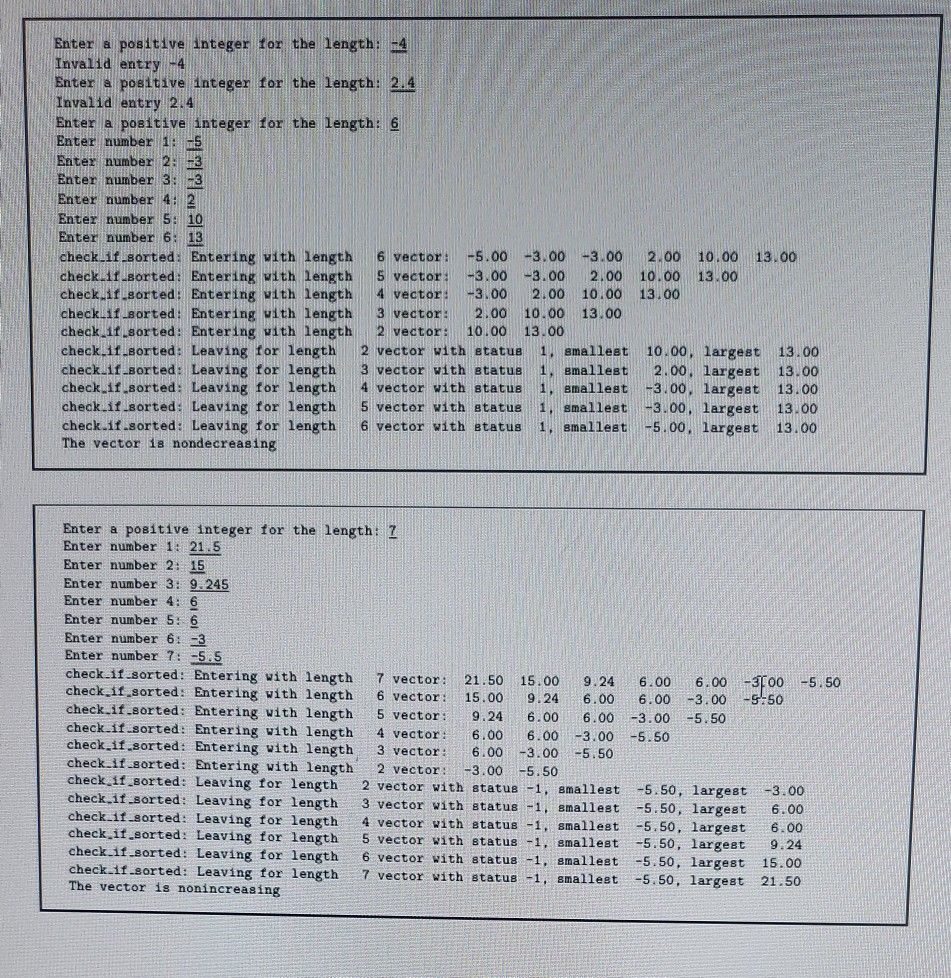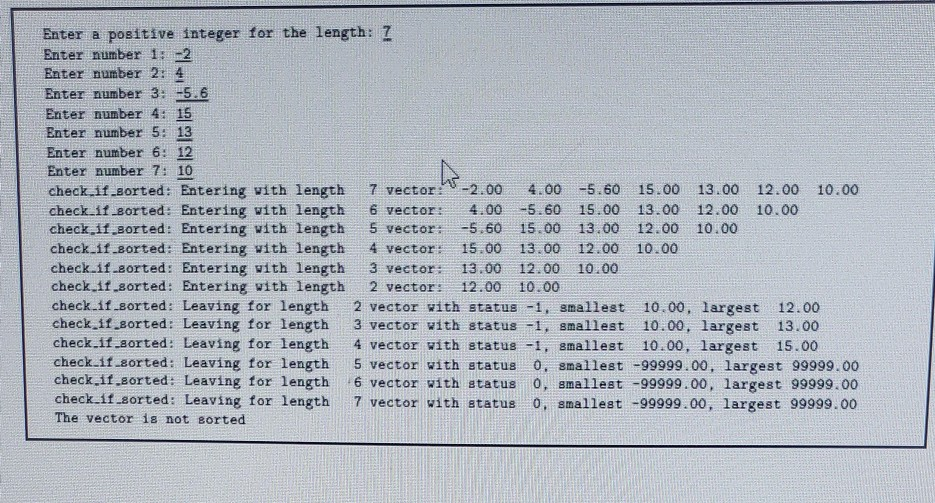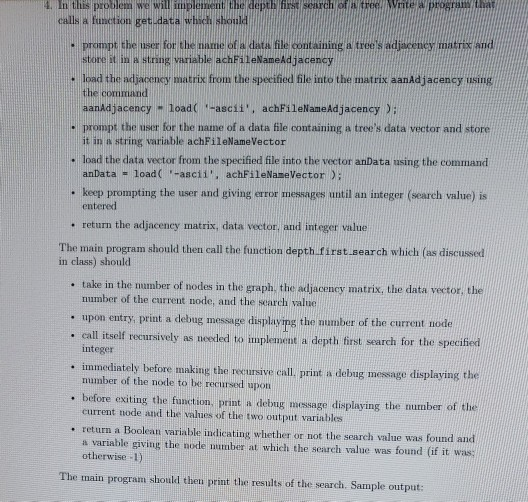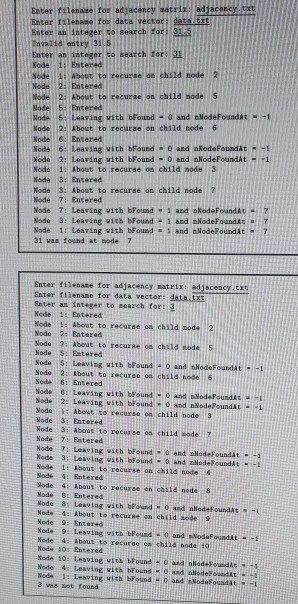2. In this problem we will modify the code for the Fibonacci sequence developed in class to give more output. Recall that the Fibonacci numbers are 0,1,1,2,3,5,8,… and can be defined recursively for n 3 as Fibonacci(n)= Fibonacci(n-1) + Fibonacci(n-2) with Fibonacci(1) = 0 and Fibonacci(2) = 1. Write a program that calls a function get positive integer which should • keep prompting the user and giving error messages until a positive integer is entered • return the resulting value The main program should then call a recursive function fibonacci which should • take in an integer n for which Fibonacci number to compute and an integer indicating how many times including the current call) the function has been called • upon entry, print a debug message displaying the values of the input variables call itself recursively as needed to calculate the specified Fibonacci number return the requested Fibonacci number and the counter of the number of calls to the fibonacci function The main program should then display the final result. Hint: Remember how to call a function that returns two variables Sample output: Enter positive integer: -2 Invalid entry – 2 Enter a positive integer 3.5 Invalid entry 3.5 Enter a positive integer: 5 Finace ter call to cepute Fibonacci 5 Fibonacci: Enter on call 2 to compute Fibonaci( 4 ) Fibonacci Enter Coll 3 to cepute Fibonacci 3 ) Fibonacel Enter call to comput. Fibonaco 2 ) REGLE Sater on call 5 to compete Fibonacci Piomacci: Enter on call to compete Fibenci 22 Fonec tor call 7 ta contrate Fibonacci 3 Fibonacci: Enter on call to compete Fibonacci (2) Fibonne Enter on all to compete Fibonace(1) P Below is a figure that might help explain the above sample output. vihen Pabananais renment ayant make Vibona Free me I ribonacotta ribanacast
3. In this problem we will investigate using recursion to determine whether ed vector is sorter (either nondecreasing or non increasing given a length 1 Vector is clearly is sorted and we will say it’s nondecreasing en g e wor easily determine if it’s sorted by comparing the two elements. Given a vector of length > 2, we can break the problem down by noting that if we knew the status and smallest and largest elements of the subvector of elements 2 through the end, we could use them und the value of the first element to determine the status of the given Vector rite a program that calls the function get vector which should call the function et positive integer (passing appropriate tring) once to obtain a value for the length of the vector use a loop to prompt the user to enter the vector one element time • return the entered vector and its length. The function get-positive integer should • take in a string input variable to use in the prompt • keep prompting the user and giving error messages using Xd) until a positive integer is entered • return the resulting value The main program should then call the function checks sorted which would • take in a vector of numbers and its length • print an entering status message giving the input variable values • call itself recursively to determine if the vector is nondecreasing nonincreasing not sorted (if it is both nondecreasing and nonincreasing call it nondecreasing) • print a “leaving a message giving the output variable values • return Da status integer that is equal to 1 if the vector is rondecreasing. -1 if it is increasing, and if it is not sorted the smallest integer present the input wtor if it is sorted or otherwise set equal to -99999 the largest integer present in the input vector if it is sorted or otherwise set equal to 99999 The main program should the print message based on the status. Sample output
Enter a positive integer for the length: -4 Invalid entry -4 Enter a positive integer for the length: 2.4 Invalid entry 2.4 Enter a positive integer for the length: 6 Enter number 1: 5 Enter number 2 -3 Enter number 3: Enter number 4: Enter number 5: 10 Enter number 6: 13 check.it sorted: Entering with length 6 vector: -5.00 -3.00 -3.00 2.00 10.00 13.00 check.11.sorted: Entering with length 5 vector: -3.00 -3.00 2.00 10.00 13.00 check if sorted! Entering with length 4 vector: -3.00 2.00 10.00 13.00 check_11_sorted: Entering with length 3 vector: 2.00 10.00 13.00 check if sorted: Entering with length 2 vector: 10.00 13.00 check.11.sorted! Leaving for length 2 vector with status 1. smallest 10.00, largest 13.00 check_if_sorted: Leaving for length 3 vector with status 1, smallest 2.00, largest 13.00 check if sorted: Leaving for length 4 vector with status -3.00, largest 13.00 check.if.sorted: Leaving for length 5 vector with status 1. smallest -3.00, largest 13.00 check. 1.sorted: Leaving for length 6 vector with status 1. smallest -5.00, largest 13.00 The vector is nondecreasing HIM smallest Enter a positive integer for the length: 7 Enter number 1: 21.5 Enter number 2: 15 Enter number 3: 9.245 Enter number 4: 6 Enter number 5: 6 Enter number 6: 3 Enter number 7: -5.5 check.it.sorted: Entering with length 7 vector: 21.50 15.00 9.24 6.00 6.00 -3700 -5.50 check it sorted: Entering with length 6 vector: 15.00 9.24 6.00 6.00 -3.00 -5:50 check.if.sorted: Entering with length 5 vector: 9.24 6.00 6.00 -3.00 -5.50 check.1f .sorted: Entering with length 4 vector: 6.00 6.00 -3.00 -5.50 check.11.sorted: Entering with length 3 vector: 6.00 -3.00 -5.50 check if sorted: Entering with length 2 vector: vector: -3.00 -3.00 -5.50 check 11 sorted: Leaving for length2 vector with status -1, smallest -5.50, large check_11_sorted: Leaving for length 3 vector with status -1, smallest -5.50, large check if sorted: Leaving for length 4 vector with status -1, smallest -5.50, large check it sorted: Leaving for length 5 vector with a 1, smallest -5.50, large 9.24 check it sorted: Leaving for length 6 vector with a smallest -5.50, largest 15.00 check.1f .sorted: Leaving for length 7 vector with status -1, smallest -5.50, largest 21.50 The vector is nonincreasing 00 00 00 tus- 00 tus
Enter a positive integer for the length: 7 Enter number 1: 2 Enter number 2: 4 Enter number 3: -5.6 Enter number 4: 15 Enter number 5: 13 Enter number 6: 12 Enter number 7: 10 check_if_sorted: Entering with length 7 vector -2.00 4.00 -5.60 15.00 13.00 12.00 10.00 check_if_sorted: Entering with length 6 vector: 4.00 -5.60 15.00 13.00 12.00 10.00 check if sorted: Entering with length 5 vector: -5.60 15.00 13.00 12.00 10.00 check if sorted: Entering with length 4 vector 15.00 13.00 12.00 10.00 check if sorted: Entering with length 3 vector: 13.00 12.00 10.00 check if sorted: Entering with length 2 vector: 12.00 10.00 check if sorted: Leaving for length 2 vector with status -1, smallest 10.00, largest 12.00 check_11_sorted: Leaving for length 3 vector with status -1, smallest 10.00, largest 13.00 check if sorted: Leaving for length 4 vector with status -1, smallest 10.00, largest 15.00 check_if_sorted: Leaving for length 5 vector with status 0. smallest -99999.00, largest 99999.00 check if sorted: Leaving for length 6 vector with s tatus o, smallest -99999.00, largest 99999.00 check_if_sorted: Leaving for length 7 vector with status 0. smallest -99999.00, largest 99999.00 The vector is not sorted

Answer to use octave or MATLAB as programs to code. do not use disp or num2str. also, do not use break or continue statements. …

We are the best freelance writing portal. Looking for online writing, editing or proofreading jobs? We have plenty of writing assignments to handle.